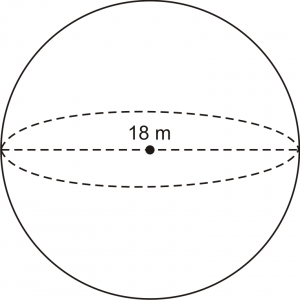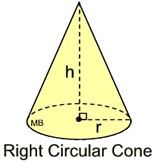## How to Calculate and Solve for the Centroid or Centre of Gravity of a SphereThe image above is a sphere and 18 m is the diameter of the sphere. In the computing of the centroid or centre of gravity of a sphere there is only one essential parameter which is the diameter of the sphere.

The formula for calculating the centroid or centre of gravity of a sphere is:

C.G. = d / 2

Where:

d is the diameter of the sphere

As always, let’s take an example:

Let’s solve an example
Find the centroid or centre of gravity of a sphere where the diameter is 18 m.

C.G. = 18 / 2
C.G. = 9

Therefore, the centroid or centre of gravity of the sphere is 9.

Nickzom Calculator – The Calculator Encyclopedia is capable of calculating the centre of gravity of a sphere at a distance from every point.

To get the answer and workings of the center of gravity or centroid of a sphere using the Nickzom Calculator – The Calculator Encyclopedia. First, you need to obtain the app.

You can get this app via any of these means:

## How to Calculate and Solve the Centre of Gravity of a Right Circular ConeThis image above is a display of what a right circular cone looks like. There is only one essential parameter for calculating the centroid or centre of gravity of a right circular cone. This parameter is the height of the cone (h).

The formula for calculating the the centroid or centre of gravity of a right circular cone is:

C.G. = h / 4

As always let’s solve an example.

Find the centroid or centre of gravity of a right circular cone where the height of the cone is 12cm.
This implies that:

h = height of the cone = 12

C.G. = 12 / 4
C.G. = 3

Therefore, the centroid or centre of gravity of the right circular cone is 3.

Nickzom Calculator – The Calculator Encyclopedia is capable of calculating the centre of gravity of a right circular cone at a distance from its base measured along the vertical axis.

To get the answer and workings to center of gravity or centroid of a right circular cone using the Nickzom Calculator – The Calculator Encyclopedia. First, you need to obtain the app.

You can get this app via any of these means:

## How to Calculate the Centre of Gravity of a Circular Sector in Statics | Mechanics

It is very possible to compute the centroid or centre of gravity of a circular sector. There are two highly important parameters one needs to know to compute the centre of gravity of a circular sector. These parameters are:

• Radius of the Sector (r)
• Semi Vertical Angle (α)

The formula for computing the centre of gravity of a circular sector is:

C.G. = 2rsinα /

Now, let’s take an example.
Let’s find the centroid or the centre of gravity of a circular sector that has a radius of 4m and a semi vertical angle of 30°.
This implies that:

r = Radius of the Sector = 4
α = Semi Vertical Angle = 30

Entering this values into the formula we have:

C.G. = 2(4)sin30° / 3(30)
C.G. = 8 . sin30° / 90
C.G. = 8 . (0.5) / 90
C.G. = 4 / 90
C.G. = 0.0444

Therefore, the centroid or centre of gravity of the circular sector is 0.0444.

Nickzom Calculator – The Calculator Encyclopedia is capable of calculating the centre of gravity of a circular sector making a semi vertical angle α at a distance from the centre of the sector measured along the central axis.

To get the answer and workings to center of gravity or centroid of a circular sector. First, you need to obtain the Nickzom Calculator – The Calculator Encyclopedia app.

## Nickzom Calculator Calculates the Center of Gravity of a Trapezium in Statics Mechanics

According to Quora,

The center of gravity of a trapezoid can be estimated by dividing the trapezoid in two triangles.

Nickzom Calculator calculates the centre of gravity of a trapezium with parallel sides a and b at a distance measured from side b.

The formula for calculating this center of gravity is:

C.G. = h (b + 2a) / 3 (b + a)

Where, a and b are the length of the parallel sides of the trapezium (b being the base length and a being the top length) whereas h is the height of the trapezium.

Let’s take for Example: Find the center of gravity or centroid of a trapezium where a is 4, b is 8 and h is 2.

This implies:

a = 4
b = 8
h = 2

C.G. = 2 (8 + 2(4)) / 3 (8 + 4)
C.G. = 2 (8 + 8) / 3 (12)
C.G. = 2 (16) / 36
C.G. = 32 / 36
C.G. = 0.89

Therefore, the center of gravity or centroid of the trapezium is 0.89.

To get the answer and workings to center of gravity or centroid of a trapezium. First, you need to obtain the Nickzom Calculator – The Calculator Encyclopedia app.

## The Calculator Encyclopedia Computes the Significant Figures of a Number

According to Wikipedia,

The significant figures (also known as the significant digits) of a number are digits that carry meaning contributing to its measurement resolution. This includes all digits except:

• All leading zeros;
• Trailing zeros when they are merely placeholders to indicate the scale of the number (exact rules are explained at identifying significant figures); and
• Spurious digits introduced, for example, by calculations carried out to greater precision than that of the original data, or measurements reported to a greater precision than the equipment supports.

Nickzom Calculator computes the significant figures of any given number.

For Example: apply 3 significant figures to 123876  and apply 2 significant figures to 0.00009872.

First, you need to obtain the Nickzom Calculator – The Calculator Encyclopedia app.
You can get this app via any of these means:

Once, you have obtained the calculator encyclopedia app, proceed to the Calculator Map, then click on Significant Figures under the Mathematics sectionOn clicking the page or activity to enter the values is displayed.From the first example: apply 3 significant figures to 123876
It implies that the number is 123876 and the number of significant figures to apply is 3.

## The Calculator Encyclopedia Calculates the Hydraulic Mean Depth or Hydraulic Radius of Flow in Open Channels | Fluid Mechanics

Hydraulic mean depth or hydraulic radius can be defined as the cross-sectional area of flow divided by wetted perimeter.

Wetted perimeter is the perimeter of the cross sectional area that is “wet”.
Hydraulic mean depth or hydraulic radius is a very important parameter in flow in open channels and fluid mechanics calculations.

The formula for computing hydraulic mean depth or hydraulic radius is:

R = A / P

Where:
R = Hydraulic Depth
A = Cross-sectional Area of Flow
P =  Wetted Perimeter

For Example:

Find the hydraulic mean depth or hydraulic radius of flow in an open channel where the cross-sectional area is 150 m2 and the wetted perimeter is 100 m.

From the example we can see that the cross-sectional area is 150 m2 and the wetted perimeter is 100 m.

R = 150 / 100
R = 1.5

Therefore, the hydraulic mean depth or hydraulic radius is 1.5 m.

Now, I would love to show you how to use Nickzom Calculator The Calculator Encyclopedia to get answers for your questions on hydraulic mean depth or hydraulic radius.

## Nickzom Calculator Calculates the Hydraulic Depth of Flow in Open Channels | Fluid Mechanics

Hydraulic depth can be defined as the cross-sectional area of flow divided by the top width of surface.

Hydraulic depth is a very crucial parameter in calculations dealing with fluid mechanics and flow in open channels.

The formula for computing hydraulic depth is:

R = A / T

Where:
R = Hydraulic Depth
A = Cross-sectional Area of Flow
T =  Top Width or Top Surface Water Width

For Example:

Find the hydraulic depth of flow in an open channel where the cross-sectional area is 120 m2 and the top width is 12 m.

From the example we can see that the cross-sectional area is 120 m2 and the top width is 12 m.

R = 120 / 12
R = 10

Therefore, the hydraulic depth is 10 m.

Now, I would love to show you how to use Nickzom Calculator The Calculator Encyclopedia to get answers for your questions on hydraulic depth.

## The Calculator Encyclopedia Calculates and Solves the Wavelength for a Wave – Particle Behaviour

According to Wikipedia,

Wave–particle duality is the concept in quantum mechanics that every particle or quantum entity may be partly described in terms not only of particles, but also of waves.

In physics, the wavelength is the spatial period of a periodic wave—the distance over which the wave’s shape repeats.

Nickzom Calculator requires two parameters to compute the wavelength of a wave or particle. These parameters are:

• Mass
• Velocity

The formula for computing the wavelength is:

λ = h / mv

Where:
λ = Wavelength
h = Planck’s Constant (6.63 x 10-34 js)
m = Mass
v = Velocity

Let’s solve an example, find the wavelength of a wave – particle with a mass of 300 Kg and a velocity of 3 x 1010 m/s.

From the example, we can see that:
m = 300
v = 3 x 1010

λ = 6.63 x 10-34 / 300 (3 x 1010)
λ = 6.63 x 10-34 / 9 x 1012
λ = 7.37 x 10-47

Therefore, the wavelength of the wave – particle (λ) is 7.37 x 10-47.

## Nickzom Calculator Calculates and Solves for Polynomials | Cubic and Quartic

According to Wikipedia,

In mathematics, a polynomial is an expression consisting of variables (also called indeterminates) and coefficients, that involves only the operations of additionsubtractionmultiplication, and non-negative integer exponents of variables.

Nickzom Calculator is the very first calculator encyclopedia to come out from Nigeria. It is capable of solving over 50,000 calculations and presenting the steps (workings). Fortunately, Nickzom Calculator can compute conception and best intercourse dates.

You can access Nickzom Calculator via any of these means:
Web – https://www.nickzom.org/calculator-plus
Android (Paid) – https://play.google.com/store/apps/details?id=org.nickzom.nickzomcalculator
Android (Free) – https://play.google.com/store/apps/details?id=com.nickzom.nickzomcalculator
Apple (Paid) – https://itunes.apple.com/us/app/nickzom-calculator/id1331162702?mt=8

Once, you have access to Nickzom Calculator proceed to the Calculator Map, click on Polynomials under Mathematics sectionFor Nickzom Calculator, there are two polynomials which are cubic and quartic.

A cubic equation or polynomial has a degree or order of 3 whereas a quartic equation or polynomial has a degree or order of 4.Let’s start with a cubic equation

## How to Calculate the Resultant of Two Vectors (Physics)

According to Math Warehouse,

The resultant vector is the vector that ‘results’ from adding two or more vectors together.

The formula for calculating the resultant of two vectors is:

R = √[P2 + Q2 + 2PQcosθ]

Where:
R = Resultant of the Two Vectors
P = Magnitude of the First Vector
Q = Magnitude of the Second Vector
θ = Inclination Angle between the Two Vectors

Let’s solve an example, find the resultant of two vectors where the first vector has a magnitude of 30 and the second vector has a magnitude of 40 with 50° has the inclination between the two vectors.

From the example above, we can see that P is 30, Q is 40 and θ is 50. Now, inserting these values into the formula we compute for the resultant of the two vectors.

R = √[(30)² + (40)² + 2(30)(40) . cos50°]
R = √[900 + 1600 + 2(1200) . (0.6427876096865394)]
R = √[900 + 1600 + 2400(0.6427876096865394)]
R = √[900 + 1600 + 1542.6902632476945]
R = √[4042.6902632476945]
R = 63.58215365373915

Therefore, the resultant of the two vectors is 63.58215365373915.

To be able to get the resultant of two vectors using Nickzom Calculator. You need to know the magnitude of the two vectors and the inclination between the two vectors.